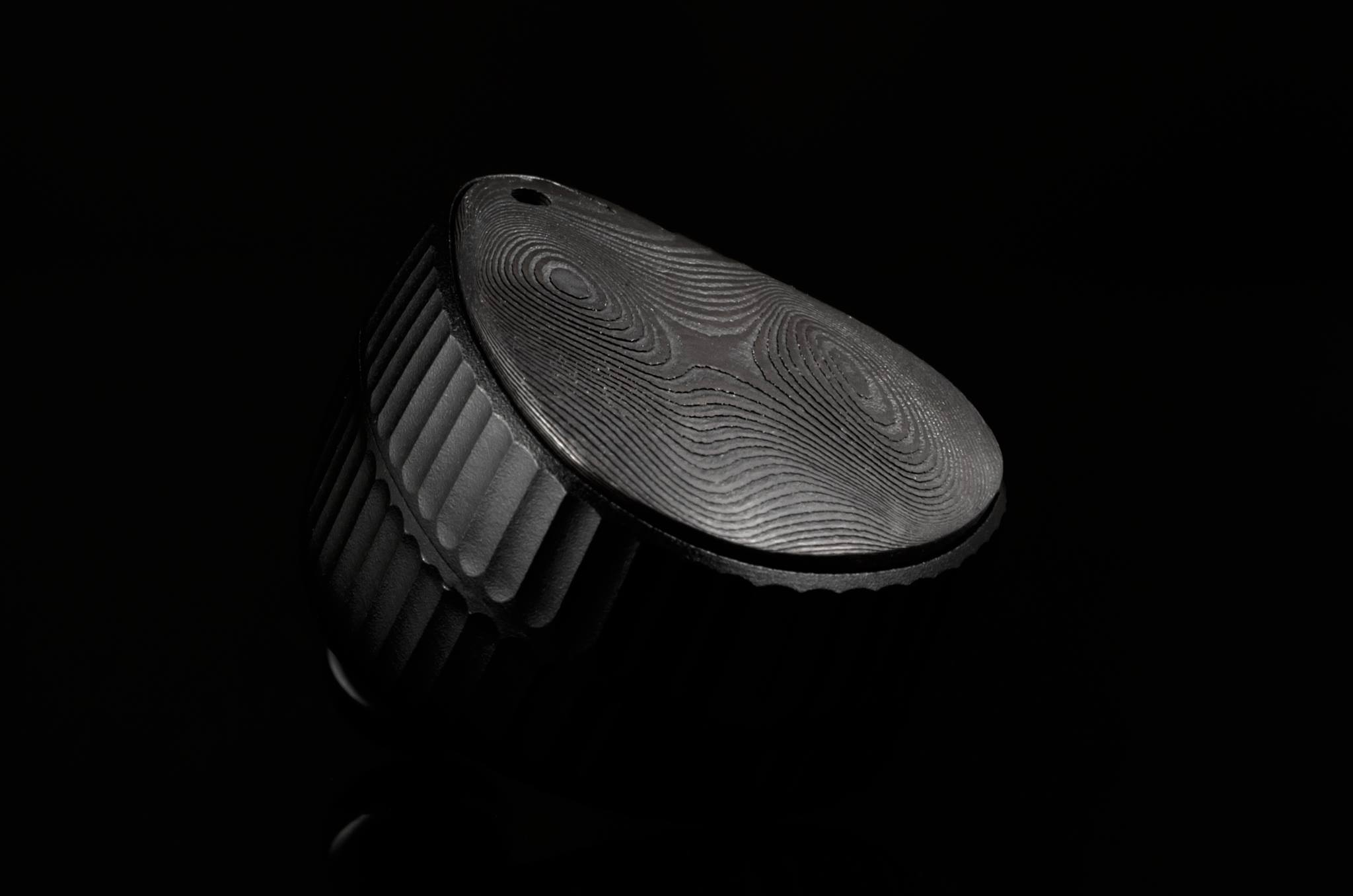# Impedance and Sensitivity

Impedance: the rated impedance. This value generally represents the lowest impedance value in the ideal state when the maximum power is reached within the approved frequency range. Because there is a margin, this value should not be higher than 20% of the lowest impedance value. This set of definitions and related test methods are based on moving coil headphones. The impedance curve of electrostatic earphones and moving iron earphones will vary greatly with frequency, and the test and definition methods may be different. From the perspective of existing products, the impedance at 1kHz or a certain frequency may be taken as the rated impedance.

If you think about the above definition further, you will find that there are many points that need to be explained. First of all, we call it impedance, which means that no matter what the principle of the speaker, it is not a pure resistance circuit. In a circuit with resistance, capacitance, and inductance, the resistance to the current in the circuit is called impedance, and the unit is ohm Ω.

Among them, R is the resistance, ωL is the inductive reactance, and 1/(ωC) is the capacitive reactance. Inductance is the obstructive effect of inductance on the current in the AC circuit, and capacitive reactance is the obstructive effect of the capacitor on the current in the AC circuit. Since it is not a physics class, the concept introduction ends here.

Although the industry test generally stipulates whether the speaker is used in the speaker or the earphone speaker, the impedance should be tested at the lowest value in a frequency range [20% deviation]. But it seems that the rated impedance of the earphones is also taken from the value under the frequency of 1kHz. Perhaps the impedance curves of moving coil and flat earphone speakers are relatively flat. Many of them will indicate that the rated impedance is obtained at 1kHz. In order not to cause too much trouble for ordinary users, it is estimated that moving coil headphones will not mark a bunch of impedance values ​​for your reference.

Impedance curve: For earphone products, the impedance curve is relatively rarely mentioned. The impedance curve of the speaker refers to the “impedance/frequency” curve in which the speaker shows different impedance values ​​when inputting signals of different frequencies. Since the impedance curve of the dynamic headphone speaker is relatively flat at 20Hz-20kHz or even higher frequencies, there are not many mentions of this indicator. On the speakers used in the soundbox, the impedance curve is an important indicator. Generally, it will have a large bump near the low frequency, and then quickly fall back [using the first trough as the rated impedance], and then as the frequency increases, The impedance may rise to vary degrees. We reproduced the test data of www.innerfidelity.com below to help you understand the difference between different earphone impedance curves.

(Graphs are taken from http://www.innerfidelity.com)

Innerfidelity puts the two curves of the relationship between frequency and impedance, as well as the relationship between frequency and phase, in one table. The phase is the phase/frequency curve, impedance is the impedance/frequency curve. The 600Ω output impedance test in the chart refers to the completion of the audio source output level of 0dBu.

What inspiration does this give us to play with headphones?

• First, the rated impedance of the earphone is the ohms at a certain point obtained by the comparison method or the substitution method. Second, the unit of moving coil, flat panel, electrostatic, moving iron earphones will change within the effective frequency [20Hz-20kHz, or higher, for example, 50kHz], instead of static.Third, the impedance curves of moving coil earphones, flat-diaphragm earphones, and moving coil earplugs are relatively flat. But the impedance curve of the moving coil large-size speaker [speaker use] is far less flat than that of the earphone.Fourth, the impedance curve of the moving iron unit is very exaggerated, and the high-frequency impedance will increase sharply when a single moving iron unit covers the full frequency band. Multi-unit moving iron is actually improving this problem, but it is still very different.Fifth, impedance is a complex number, which is related to signal frequency and phase.

Sixth, the impedance cannot be equal to the external resistance, especially earphones with huge changes in the impedance curve, which may bring a very strange sense of hearing.